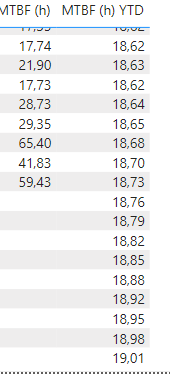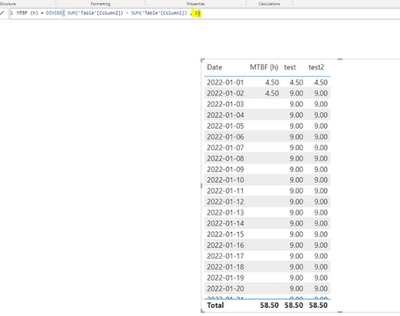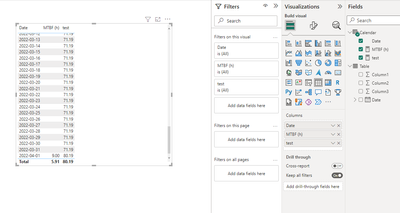cancel
Showing results for
Did you mean:Frequent Visitor

## TOTALYTD accumulating when it's not supposed to

Hello, I'm using the TOTALYTD quick measure to calculate the year-to-date cummulative value of my MTBF metric but when i have no MTBF values, I was expecting the MTBF (h) YTD stay the same, but instead it's showing me increasing values and I don't know why or where they come from, any clue?

Here is the measure:

``````MTBF (h) YTD =
TOTALYTD([MTBF (h)],'Calendar'[Date])``````

Here's what's happening for my 'Calendar' [Date] selection1 ACCEPTED SOLUTIONCommunity Support

Hi , @killjoy

Oh, sorry for not detailed explanation. Not for all the DIVIDE() function is not support for the TOTALYTD() function, just when the denominator is not dynamic. If the denominator is static , it works good,like this:

test2 = TOTALYTD([MTBF (h)],'Calendar'[Date])For this reason, the actual logic is like this:

``````CALCULATE(DIVIDE( SUM('Table'[Column2]) - SUM('Table'[Column1]) , 2) ,  DATESYTD('Date'[Date])  )

``````

If the denominators are different, then in different filtering contexts, the sum will not be performed but the average will be performed.

And the bottom layer of TOTAL YTD() is also implemented using the FILTER () function, so it is recommended that you use the above method for some special cases.

Thank you for your time and sharing, and thank you for your support and understanding of PowerBI!

Best Regards,

Aniya Zhang

If this post helps, then please consider Accept it as the solution to help the other members find it more quickly

10 REPLIES 10Community Support

Hi , @killjoy

Oh, sorry for not detailed explanation. Not for all the DIVIDE() function is not support for the TOTALYTD() function, just when the denominator is not dynamic. If the denominator is static , it works good,like this:

test2 = TOTALYTD([MTBF (h)],'Calendar'[Date])For this reason, the actual logic is like this:

``````CALCULATE(DIVIDE( SUM('Table'[Column2]) - SUM('Table'[Column1]) , 2) ,  DATESYTD('Date'[Date])  )

``````

If the denominators are different, then in different filtering contexts, the sum will not be performed but the average will be performed.

And the bottom layer of TOTAL YTD() is also implemented using the FILTER () function, so it is recommended that you use the above method for some special cases.

Thank you for your time and sharing, and thank you for your support and understanding of PowerBI!

Best Regards,

Aniya Zhang

If this post helps, then please consider Accept it as the solution to help the other members find it more quicklyCommunity Support

Hi , @killjoy

I test the DIVIDE() function, in my side , when i use the TOTALYTD() function, it return the same as yours.

For this , you can try to use this method:
(1)This is my test data:(2)I create a measure as [MTBF(h)] like you:

``MTBF (h) = DIVIDE( SUM('Table'[Column2]) - SUM('Table'[Column1]) , SUM('Table'[Column3]))``

(3)We can create a measure like this to get the right YTD value:

``````test = var _cur_date = MAX('Calendar'[Date])
var _t = SUMMARIZE(ALL('Calendar') , 'Calendar'[Date] , "MTBF (h)", [MTBF (h)])
var _t2 = FILTER( _t , [Date]<=_cur_date && YEAR([Date]) = YEAR(_cur_date))
return
SUMX(_t2,[MTBF (h)])``````

(4)Then we can put the fields we need on the visual and we can meet your need:Thank you for your time and sharing, and thank you for your support and understanding of PowerBI!

Best Regards,

Aniya Zhang

If this post helps, then please consider Accept it as the solution to help the other members find it more quicklyFrequent Visitor

Thank you so much for your help, but does that mean the TOTALYTD does not work if I use the DIVIDE()?  I have another measure similar to MTBF that usess DIVIDE and the TOTALYTD there is working as I expected.Frequent Visitor

I see, but in the example you have you're using the sum of values from a column, and I'm using a measure as the <expression> for TOTALYTD.Solution Sage

Even If I switch my simple SUM with a measure (containing SUM), result is the same:### If my answer was helpful please give me a Kudos or even accept as a Solution.Frequent Visitor

I don't know what I'm doing wrong then... My "MTBF(h)" is just a divide with a subtraction :

``````DIVIDE(
[#M1] - [#M2] - [#M3] - [#M4],
[#M5]
)``````Solution Sage

How do you calculate M1, M2...?

Example you have above should not make any problems.

### If my answer was helpful please give me a Kudos or even accept as a Solution.Frequent Visitor

They're all calculated in a similar way:

``````M1 =
SUM(Table1[ColumnA])``````

The rest are as like:

``````# M2,M3,M4.. =
CALCULATE(
SUM(Table2[ColumnB]),
'Table3'[ColumnC] in {'x','y','z'}
)``````Solution Sage

Frankly only thing which I have in mind is , that one of the "M" measures contains values. That would explain why you see some changes.
Otherwise try to debug the DEVIDE step by step.

### If my answer was helpful please give me a Kudos or even accept as a Solution.Solution Sage

Hi @killjoy ,

I think the cause might be done by the expression in the other measure yoou have

`[MTBF (h)]`

I used the same example with simple sum and everything works well:### If my answer was helpful please give me a Kudos or even accept as a Solution.